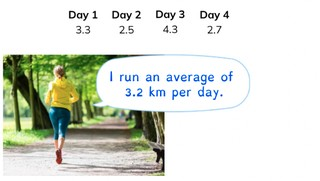Determining the average with decimal numbers

# Determining the average with decimal numbers

Students learn to determine the average with decimal numbers.

No account needed.8,000 schools use Gynzy92,000 teachers use Gynzy1,600,000 students use Gynzy

## General

The students learn to determine the average with decimal numbers.

## Standards

CCSS.Math.Content.6.SP.B.5.C

## Learning objective

Students will be able to determine the average of multiple decimal numbers.

## Introduction

Have the students solve the division problems. Discuss how to solve them afterwords if this is necessary.

## Instruction

Explain that when determining the average with decimal numbers you begin by adding the numbers together. Show that you add the numbers 3.1 | 4.3 | 11.2 togehter (3.1 + 4.3 + 11.2 = 18.6). Next you divide the total by the number of numbers that you added together to determine the average (18.6 ÷ 3 = 6.2). You can practice solving the following problem together with the students. You may remove the cover over the speech bubble to show the steps, as a reminder for the students. The students determine the average in the following problem on their own. Show the table. Explain that you are going to determine the average grade for social studies. First add the numbers in the table together (9 + 8.3 + 10 = 27.3). Now divide the total by the number of numbers (27.3 ÷ 3 = 9.1). Next you have the students determine the average grade for spelling.

Check whether the students can determine the average with decimal numbers, by asking the following question:
- What steps do you take to determine the average with multiple decimal numbers?

## Quiz

The students test their understanding of determining the average with decimal numbers through ten exercises. In each of these exercises, they must determine the average of two or more decimal numbers. For some of the exercises the students must use numbers from a table, and other exercises are story problems.

## Closing

Discuss once again the importance of determining the average of multiple decimal numbers. As a closing activity have the students run to the traffic cones in the classroom with decimal numbers on them. They run to each traffic cone, adding the numbers together as they go. After a minute is up, they stop. Do this three times. Then they must determine the average of these three numbers.

## Teaching tips

Have students that have difficulty with determining the average with decimal numbers first practice with the division table of 2. Have them add two numbers together and then divide evenly between two students. For this you can use concrete materials (blocks). Next they can practice determining the average of whole numbers.

### The online teaching platform for interactive whiteboards and displays in schools

• Save time building lessons

• Manage the classroom more efficiently

• Increase student engagement